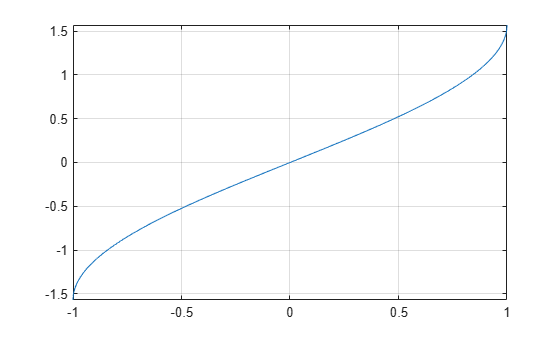# asin

Symbolic inverse sine function

## Syntax

``asin(X)``

## Description

example

````asin(X)` returns the inverse sine function (arcsine function) of `X`. All angles are in radians. For real values of `X` in the interval `[-1,1]`, `asin(X)` returns the values in the interval `[-pi/2,pi/2]`.For real values of `X` outside the interval `[-1,1]` and for complex values of `X`, `asin(X)` returns complex values with the real parts in the interval `[-pi/2,pi/2]`. ```

## Examples

### Inverse Sine Function for Numeric and Symbolic Arguments

Depending on its arguments, `asin` returns floating-point or exact symbolic results.

Compute the inverse sine function for these numbers. Because these numbers are not symbolic objects, `asin` returns floating-point results.

`A = asin([-1, -1/3, -1/2, 1/4, 1/2, sqrt(3)/2, 1])`
```A = -1.5708 -0.3398 -0.5236 0.2527 0.5236 1.0472 1.5708```

Compute the inverse sine function for the numbers converted to symbolic objects. For many symbolic (exact) numbers, `asin` returns unresolved symbolic calls.

`symA = asin(sym([-1, -1/3, -1/2, 1/4, 1/2, sqrt(3)/2, 1]))`
```symA = [ -pi/2, -asin(1/3), -pi/6, asin(1/4), pi/6, pi/3, pi/2]```

Use `vpa` to approximate symbolic results with floating-point numbers:

`vpa(symA)`
```ans = [ -1.5707963267948966192313216916398,... -0.33983690945412193709639251339176,... -0.52359877559829887307710723054658,... 0.25268025514207865348565743699371,... 0.52359877559829887307710723054658,... 1.0471975511965977461542144610932,... 1.5707963267948966192313216916398]```

### Plot Inverse Sine Function

Plot the inverse sine function on the interval from -1 to 1.

```syms x fplot(asin(x),[-1 1]) grid on```### Handle Expressions Containing Inverse Sine Function

Many functions, such as `diff`, `int`, `taylor`, and `rewrite`, can handle expressions containing `asin`.

Find the first and second derivatives of the inverse sine function:

```syms x diff(asin(x), x) diff(asin(x), x, x)```
```ans = 1/(1 - x^2)^(1/2) ans = x/(1 - x^2)^(3/2)```

Find the indefinite integral of the inverse sine function:

`int(asin(x), x)`
```ans = x*asin(x) + (1 - x^2)^(1/2)```

Find the Taylor series expansion of `asin(x)`:

`taylor(asin(x), x)`
```ans = (3*x^5)/40 + x^3/6 + x```

Rewrite the inverse sine function in terms of the natural logarithm:

`rewrite(asin(x), 'log')`
```ans = -log((1 - x^2)^(1/2) + x*1i)*1i```

## Input Arguments

collapse all

Input, specified as a symbolic number, variable, expression, or function, or as a vector or matrix of symbolic numbers, variables, expressions, or functions.